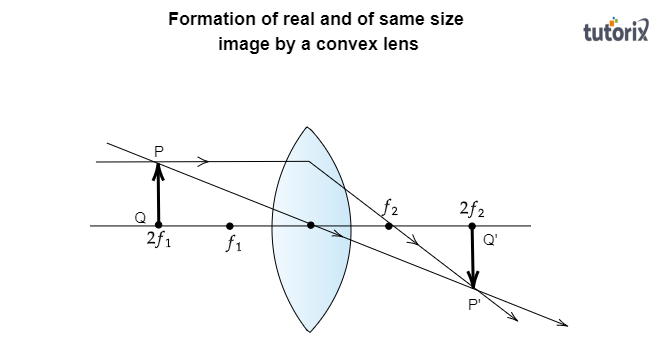# Where should an object be placed in front of a convex lens to get a real image of the size of the object?(a) At the principal focus of the lens(b) At twice the focal length(c) At infinity(d) Between the optical center of the lens and its principal focus.

The correct answer is: $(b)$ At twice the focal length

Formation of the real image by a convex lens$\rightarrow$

When an object is placed at a distance greater than its focal length$(f)$ from a convex lens, a real image is formed

Formation of images of the same size$\rightarrow$

When the object is placed at the center of the curvature$(2f)$ of the convex lens, a real image of the same size is formed at the center of the second curvature of the convex lens.

The given diagram below shows the formation of a real image of the size of the object. in the diagram, the object PQ is placed at the center $2f_1$  of the curvature of the convex lens, and the real image of the same size $P'Q'$ is formed at the center $(2f_2)$ of another curvature.

Here, $2f_1=2f_2$                                 [twice of the focal length $(f_1)$]Conclusion$\rightarrow$

Therefore, an object should be placed at double the focal length in front of a convex lens to get a real image of the size of the object.

Hence, option (b) is correct.

Updated on: 10-Oct-2022

957 Views